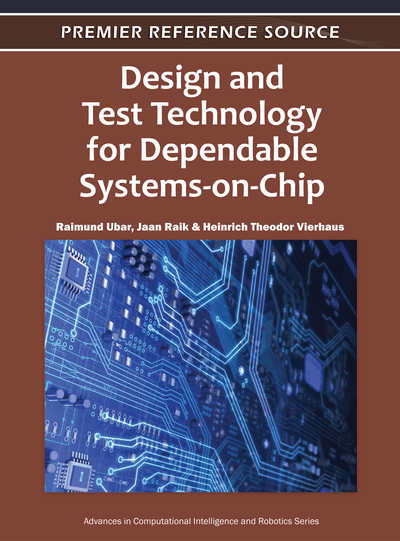# Low Power Testing

Zdenek Kotásek (Brno University of Technology, Czech Republic) and Jaroslav Škarvada (Brno University of Technology, Czech Republic)
DOI: 10.4018/978-1-60960-212-3.ch018

## Abstract

Portable computer systems and embedded systems are examples of electronic devices which are powered from batteries; therefore, they are designed with the goal of low power consumption. Low power consumption becomes important not only during normal operational mode, but during test application as well when switching activity is higher than in normal mode. In this chapter, a survey of basic concepts and methodologies from the area of low power testing is provided. It is explained here how power consumption is related to switching activities during test application. The concepts of static and dynamic power consumption are discussed together with metrics which can be possibly used to evaluate power consumption. The survey of methods, the goal of which is to reduce dynamic power consumption during test application, is then provided followed by a short survey of power-constrained test scheduling methods.
Chapter Preview
Top

## Introduction

Power consumption of an electronic component is different for various technologies and platforms. CMOS technology is a dominant technology used in VLSI design (Nicolici & Al-Hashimi, 2003). A CMOS gate structure can be seen in Figure 1.

Two important characteristics of CMOS devices are high noise immunity and low static power consumption. Significant power is only drawn while the transistors in a CMOS device are switching between on and off states. CMOS devices do not produce as much waste heat as other forms of logic, for example transistor-transistor logic (TTL) or NMOS logic which uses all n-channel devices without p-channel devices. CMOS also allows a high density of logic functions on a chip. It was primarily this reason why CMOS won the race in the eighties and became the most used technology to be implemented in VLSI chips. Consequently, to develop methodologies which allow us to reduce power consumption during test application became a necessity.

The diagram of power consumption during test application is shown in Figure 2. Test vectors V1 – V7 are applied at t1 – t7, ti = i/f; i = (1;7) is a pulse sequential index, f is the frequency of clock pulses. In the figure, the test per clock (TPC) strategy is demonstrated here in which one test vector is applied by one clock pulse and a response is gained. If the test is applied through a scan register then the test per scan (TPS) strategy is used. During one test step several clock pulses are applied.

Power consumption value can be evaluated by means of the following formula (Raghunathan, Jha & Dey, 1998; Roy & Prasad, 2000):p(t) = ps(t) +pd(t)(1) where p(t) represents power consumption at time t, ps(t) which is the static power consumption, while pd(t) is the dynamic power consumption.

It is evident that switching activity plays an important role in considerations about power consumption of electronic component. The objective of this chapter is to primarily explain the relation between the range of switching activity in VLSI components and power consumption. It is a well known fact that power consumption is higher during test application than during normal operation, therefore special attention is paid to techniques which have as its goal to reduce power consumption during test application (Girard, Nicolici & Wen, 2010).

### Static Power Consumption Ps

The value of ps(t) is not changing in time, therefore it can be marked as Ps. It can be enumerated by means of the following formula:

(2)

In (2), the symbols have the following meaning: Idiode – reversal current between diffusion area and substrate, Isubtreshold – leakage current (see formula 3), Udd – supply voltage

(3)

In (3), the constants depend on the technology level of transistors used in the design. The meaning of the symbols is as follows: Weff - effective width of transistor channel, Uin - the value of input voltage, UT - the value of threshold voltage. The value of Ps increases exponentially with a decreasing UT value. In most technologies used in 2008 - 2009, the value of Ps is not more than 50% of total power consumption value. In a significant number of electronic components produced in older technologies, it is even less--not more than 10%. The Ps value is constant in time and does not depend on input signals (see Ps in Figure 2).

## Complete Chapter List

Search this Book:
Reset